## Kiepert's Hyperbola

A curve which is related to the solution of Lemoine's Problem and its generalization to Isosceles Triangles constructed on the sides of a given Triangle. The Vertices of the constructed Triangles are(1)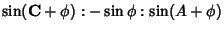(2)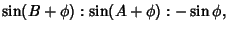(3)

where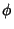is the base Angle of the Isosceles Triangle. Kiepert showed that the lines connecting the Vertices of the given Triangle and the corresponding peaks of the Isosceles Triangles Concur. The Trilinear Coordinates of the point of concurrence are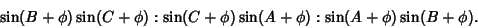(4)

The locus of this point as the base Angle varies is given by the curve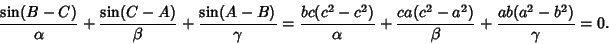(5)

Writing the Trilinear Coordinates as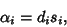(6)

where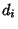is the distance to the side oppositeof length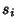and using the Point-Line Distance Formula withwritten as,(7)

whereandgives the Formula(8)(9)

Bringing this equation over a common Denominator then gives a quadratic inand, which is a Conic Section (in fact, a Hyperbola). The curve can also be written as, as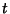varies over.Kiepert's hyperbola passes through the triangle's Centroid(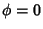), Orthocenter(), Vertices(ifandif),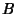(),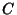(), Fermat Point(), second Isogonic Center(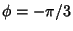), Isogonal Conjugate of the Brocard Midpoint (), and Brocard's Third Point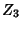(), where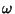is the Brocard Angle (Eddy and Fritsch 1994, p. 193).

The Asymptotes of Kiepert's hyperbola are the Simson Lines of the intersections of the Brocard Axis with the Circumcircle. Kiepert's hyperbola is a Rectangular Hyperbola. In fact, all nondegenerate conics through the Vertices and Orthocenter of a Triangle are Rectangular Hyperbolas the centers of which lie halfway between the Isogonic Centers and on the Nine-Point Circle. The Locus of centers of these Hyperbolas is the Nine-Point Circle.

The Isogonal Conjugate curve of Kiepert's hyperbola is the Brocard Axis. The center of the Incircle of the Triangle constructed from the Midpoints of the sides of a given Triangle lies on Kiepert's hyperbola of the original Triangle.

See also Brocard Angle, Brocard Axis, Brocard Points, Centroid (Triangle), Circumcircle, Isogonal Conjugate, Isogonic Centers, Isosceles Triangle, Lemoine's Problem, Nine-Point Circle, Orthocenter, Simson Line

References

Casey, J. A Treatise on the Analytical Geometry of the Point, Line, Circle, and Conic Sections, Containing an Account of Its Most Recent Extensions with Numerous Examples, 2nd rev. enl. ed. Dublin: Hodges, Figgis, & Co., 1893.

Eddy, R. H. and Fritsch, R. The Conics of Ludwig Kiepert: A Comprehensive Lesson in the Geometry of the Triangle.'' Math. Mag. 67, 188-205, 1994.

Kelly, P. J. and Merriell, D. Concentric Polygons.'' Amer. Math. Monthly 71, 37-41, 1964.

Mineuer, A. Sur les asymptotes de l'hyperbole de Kiepert.'' Mathesis 49, 30-33, 1935.

Rigby, J. F. A Concentrated Dose of Old-Fashioned Geometry.'' Math. Gaz. 57, 296-298, 1953.

Vandeghen, A. Some Remarks on the Isogonal and Cevian Transforms. Alignments of Remarkable Points of a Triangle.'' Amer. Math. Monthly 72, 1091-1094, 1965.